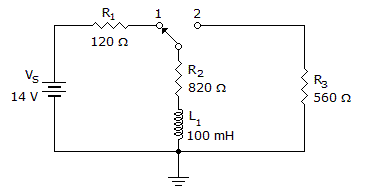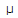# Electronics - Inductors - Discussion

### Discussion :: Inductors - General Questions (Q.No.30)

30.If the switch is moved to position 1 in the given circuit, how long will it take for the current to reach its maximum value?

 [A]. 72.5s [B]. 532s [C]. 610s [D]. 833s

Explanation:

No answer description available for this question.

 Prudvi said: (May 31, 2011) Please tel me how to solve it!

 Rashid Call3135791000 said: (Aug 7, 2011) It is RL circuit, we use Vs=R + Inductor.

 Priya said: (Nov 23, 2011) Time constant for RL circuit is t = L/R t=0.1/940=0.1063829ms 5 time time constants it will to reach its max value. t = 5*0.1063829ms = 532 us.

 Kiran said: (Nov 27, 2012) How we had taken resistance value?

 Tushar Sharma said: (May 28, 2013) Time constant for LR circuit is t = L/R. Now R=120+820 = 940R. L = 100mH. So t = L/R =0.1063829ms. To reach maximum i.e. 100% value; it will take 5 time constants, 5 Time constants. 1T 63.2% 2T 86.4% 3T 94.9% 4T 98.1% 5T 100% So, T = 5*0.1063829ms = 532us.

 Yateesh Kumar Vc said: (Mar 3, 2016) On which basis t is multiplied by 5. I didn't please solve this problem.

 Balu said: (Apr 28, 2017) Because the current will have reached 99.5% of the steady state value after 5 time constants.

 Pritam said: (Apr 22, 2020) At position 2 inductor is discharging. Before coming to position 1 inductor is completely discharged. Its general formula for complete charging of capacitor and inductor 5T. 5*L/R=5*0.1/940=532us.